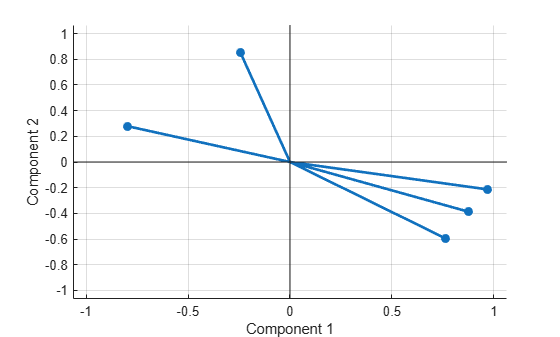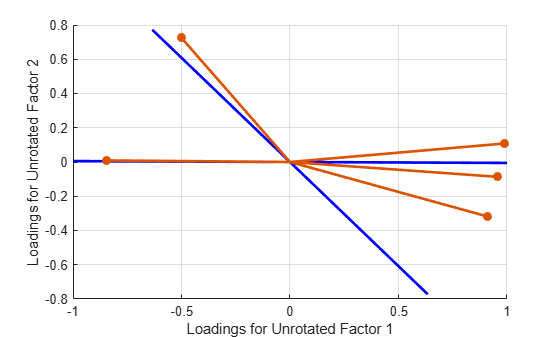Documentation

# factoran

Factor analysis

## Syntax

```lambda = factoran(X,m) [lambda,psi] = factoran(X,m) [lambda,psi,T] = factoran(X,m) [lambda,psi,T,stats] = factoran(X,m) [lambda,psi,T,stats,F] = factoran(X,m) [...] = factoran(...,param1,val1,param2,val2,...) ```

## Description

`lambda = factoran(X,m)` returns the maximum likelihood estimate, `lambda`, of the factor loadings matrix, in a common factor analysis model with `m` common factors. `X` is an `n`-by-`d` matrix where each row is an observation of `d` variables. The `(i,j)`th element of the `d`-by-`m` matrix `lambda` is the coefficient, or loading, of the `j`th factor for the `i`th variable. By default, `factoran` calls the function `rotatefactors` to rotate the estimated factor loadings using the `'varimax'` option.

`[lambda,psi] = factoran(X,m)` also returns maximum likelihood estimates of the specific variances as a column vector `psi` of length `d`.

`[lambda,psi,T] = factoran(X,m)` also returns the `m`-by-`m` factor loadings rotation matrix `T`.

`[lambda,psi,T,stats] = factoran(X,m)` also returns a structure `stats` containing information relating to the null hypothesis, H0, that the number of common factors is `m`. `stats` includes the following fields:

FieldDescription
`loglike`

Maximized log-likelihood value

`dfe`

Error degrees of freedom = `((d-m)^2 - (d+m))/2`

`chisq`

Approximate chi-squared statistic for the null hypothesis

`p`

Right-tail significance level for the null hypothesis

`factoran` does not compute the `chisq` and `p` fields unless `dfe` is positive and all the specific variance estimates in `psi` are positive (see Heywood Case below). If `X` is a covariance matrix, then you must also specify the `'nobs'` parameter if you want `factoran` to compute the `chisq` and `p` fields.

`[lambda,psi,T,stats,F] = factoran(X,m)` also returns, in `F`, predictions of the common factors, known as factor scores. `F` is an `n`-by-`m` matrix where each row is a prediction of `m` common factors. If `X` is a covariance matrix, `factoran` cannot compute `F`. `factoran` rotates `F` using the same criterion as for `lambda`.

`[...] = factoran(...,param1,val1,param2,val2,...)` enables you to specify optional parameter name/value pairs to control the model fit and the outputs. The following are the valid parameter/value pairs.

ParameterValue
`'xtype'`

Type of input in the matrix `X`. `'xtype'` can be one of:

`'data'`

Raw data (default)

`'covariance'`

Positive definite covariance or correlation matrix

`'scores'`

Method for predicting factor scores. `'scores'` is ignored if `X` is not raw data.

```'wls' 'Bartlett'```

Synonyms for a weighted least-squares estimate that treats `F` as fixed (`default`)

```'regression' 'Thomson'```

Synonyms for a minimum mean squared error prediction that is equivalent to a ridge regression

`'start' `

Starting point for the specific variances `psi` in the maximum likelihood optimization. Can be specified as:

`'random'`

Chooses `d` uniformly distributed values on the interval [0,1].

`'Rsquared'`

Chooses the starting vector as a scale factor times `diag(inv(corrcoef(X)))` (default). For examples, see Jöreskog .

Positive integer

Performs the given number of maximum likelihood fits, each initialized as with `'random'`. `factoran` returns the fit with the highest likelihood.

Matrix

Performs one maximum likelihood fit for each column of the specified matrix. The `i`th optimization is initialized with the values from the `i`th column. The matrix must have `d` rows.

`'rotate'`

Method used to rotate factor loadings and scores. `'rotate'` can have the same values as the `'Method'` parameter of `rotatefactors`. See the reference page for `rotatefactors` for a full description of the available methods.

`'none'`

Performs no rotation.

`'equamax'`

Special case of the orthomax rotation. Use the `'normalize'`, `'reltol'`, and `'maxit'` parameters to control the details of the rotation.

`'orthomax'`

Orthogonal rotation that maximizes a criterion based on the variance of the loadings.

Use the `'coeff'`, `'normalize'`, `'reltol'`, and `'maxit'` parameters to control the details of the rotation.

`'parsimax'`

Special case of the orthomax rotation (default). Use the `'normalize'`, `'reltol'`, and '`maxit`' parameters to control the details of the rotation.

`'pattern'`

Performs either an oblique rotation (the default) or an orthogonal rotation to best match a specified pattern matrix. Use the `'type'` parameter to choose the type of rotation. Use the `'target'` parameter to specify the pattern matrix.

`'procrustes'`

Performs either an oblique (the default) or an orthogonal rotation to best match a specified target matrix in the least squares sense.

Use the `'type'` parameter to choose the type of rotation. Use `'target'` to specify the target matrix.

`'promax'`

Performs an oblique procrustes rotation to a target matrix determined by `factoran` as a function of an orthomax solution.

Use the `'power'` parameter to specify the exponent for creating the target matrix. Because `'promax'` uses `'orthomax'` internally, you can also specify the parameters that apply to `'orthomax'`.

`'quartimax'`

Special case of the orthomax rotation (default). Use the `'normalize'`, `'reltol'`, and '`maxit`' parameters to control the details of the rotation.

`'varimax'`

Special case of the orthomax rotation (default). Use the `'normalize'`, `'reltol'`, and `'maxit'` parameters to control the details of the rotation.

Function

Function handle to rotation function of the form

```[B,T] = myrotation(A,...)```

where `A` is a `d`-by-`m` matrix of unrotated factor loadings, `B` is a `d`-by-`m` matrix of rotated loadings, and `T` is the corresponding `m`-by-`m` rotation matrix.

Use the `factoran` parameter `'userargs'` to pass additional arguments to this rotation function. See User-Defined Rotation Function.

`'coeff'`

Coefficient, often denoted as γ, defining the specific `'orthomax'` criterion. Must be from `0` to `1`. The value `0` corresponds to quartimax, and `1` corresponds to varimax. Default is `1`.

`'normalize'`

Flag indicating whether the loading matrix should be row-normalized (1) or left unnormalized (0) for `'orthomax'` or `'varimax'` rotation. Default is 1.

`'reltol'`

Relative convergence tolerance for `'orthomax'` or `'varimax'` rotation. Default is `sqrt(eps)`.

`'maxit'`

Iteration limit for `'orthomax'` or `'varimax'` rotation. Default is `250`.

`'target'`

Target factor loading matrix for `'procrustes'` rotation. Required for `'procrustes'` rotation. No default value.

`'type'`

Type of `'procrustes'` rotation. Can be `'oblique'` (default) or `'orthogonal'`.

`'power'`

Exponent for creating the target matrix in the `'promax'` rotation. Must be ≥ `1`. Default is `4`.

`'userargs'`

Denotes the beginning of additional input values for a user-defined rotation function. `factoran` appends all subsequent values, in order and without processing, to the rotation function argument list, following the unrotated factor loadings matrix `A`. See User-Defined Rotation Function.

`'nobs'`

If `X` is a covariance or correlation matrix, indicates the number of observations that were used in its estimation. This allows calculation of significance for the null hypothesis even when the original data are not available. There is no default. `'nobs'` is ignored if `X` is raw data.

`'delta'`

Lower bound for the specific variances `psi` during the maximum likelihood optimization. Default is `0.005`.

`'optimopts'`

Structure that specifies control parameters for the iterative algorithm the function uses to compute maximum likelihood estimates. Create this structure with the function `statset`. Enter `statset('factoran')` to see the names and default values of the parameters that `factoran` accepts in the `options` structure. See the reference page for `statset` for more information about these options.

## Examples

collapse all

`load carbig`

Define the variable matrix.

```X = [Acceleration Displacement Horsepower MPG Weight]; X = X(all(~isnan(X),2),:);```

Estimate the factor loadings using a minimum mean squared error prediction for a factor analysis with two common factors.

```[Lambda,Psi,T,stats,F] = factoran(X,2,'scores','regression'); inv(T'*T); % Estimated correlation matrix of F, == eye(2) Lambda*Lambda' + diag(Psi); % Estimated correlation matrix Lambda*inv(T); % Unrotate the loadings F*T'; % Unrotate the factor scores```

Create biplot of two factors.

`biplot(Lambda,'LineWidth',2,'MarkerSize',20)``[Lambda,Psi,T] = factoran(cov(X),2,'xtype','cov')`
```Lambda = 5×2 -0.2432 -0.8500 0.8773 0.3871 0.7618 0.5930 -0.7978 -0.2786 0.9692 0.2129 ```
```Psi = 5×1 0.2184 0.0804 0.0680 0.2859 0.0152 ```
```T = 2×2 0.9476 0.3195 0.3195 -0.9476 ```
`% [Lambda,Psi,T] = factoran(corrcoef(X),2,'xtype','cov')`

Although the estimates are the same, the use of a covariance matrix rather than raw data doesn't let you request scores or significance level.

Use promax rotation.

```[Lambda,Psi,T,stats,F] = factoran(X,2,'rotate','promax',... 'powerpm',4); inv(T'*T) % Estimated correlation of F, ```
```ans = 2×2 1.0000 -0.6391 -0.6391 1.0000 ```
``` % no longer eye(2) Lambda*inv(T'*T)*Lambda'+diag(Psi) % Estimated correlation of X```
```ans = 5×5 1.0000 -0.5424 -0.6893 0.4309 -0.4167 -0.5424 1.0000 0.8979 -0.8078 0.9328 -0.6893 0.8979 1.0000 -0.7730 0.8647 0.4309 -0.8078 -0.7730 1.0000 -0.8326 -0.4167 0.9328 0.8647 -0.8326 1.0000 ```

Plot the unrotated variables with oblique axes superimposed.

```invT = inv(T); Lambda0 = Lambda*invT; figure() line([-invT(1,1) invT(1,1) NaN -invT(2,1) invT(2,1)], ... [-invT(1,2) invT(1,2) NaN -invT(2,2) invT(2,2)], ... 'Color','r','linewidth',2) grid on hold on biplot(Lambda0,'LineWidth',2,'MarkerSize',20) xlabel('Loadings for unrotated Factor 1') ylabel('Loadings for unrotated Factor 2')```Plot the rotated variables against the oblique axes.

```figure() biplot(Lambda,'LineWidth',2,'MarkerSize',20)```Syntax for passing additional arguments to a user-defined rotation function:

```[Lambda,Psi,T] = ... factoran(X,2,'rotate',@myrotation,'userargs',1,'two'); ```

collapse all

### Factor Analysis Model

`factoran` computes the maximum likelihood estimate (MLE) of the factor loadings matrix Λ in the factor analysis model

`$x=\mu +\Lambda f+e$`

where x is a vector of observed variables, μ is a constant vector of means, Λ is a constant d-by-m matrix of factor loadings, f is a vector of independent, standardized common factors, and e is a vector of independent specific factors. x, μ, and e are of length d. f is of length m.

Alternatively, the factor analysis model can be specified as

`$\mathrm{cov}\left(x\right)=\Lambda {\Lambda }^{T}+\Psi$`

where $\Psi =\mathrm{cov}\left(e\right)$ is a d-by-d diagonal matrix of specific variances.

## Tips

### Observed Data Variables

The variables in the observed data matrix `X` must be linearly independent, i.e., `cov(X)` must have full rank, for maximum likelihood estimation to succeed. `factoran` reduces both raw data and a covariance matrix to a correlation matrix before performing the fit.

`factoran` standardizes the observed data `X` to zero mean and unit variance before estimating the loadings `lambda`. This does not affect the model fit, because MLEs in this model are invariant to scale. However, `lambda` and `psi` are returned in terms of the standardized variables, i.e., `lambda*lambda'+diag(psi)` is an estimate of the correlation matrix of the original data `X` (although not after an oblique rotation). See Estimate and Plot Factor Loadings and User-Defined Rotation Function.

### Heywood Case

If elements of `psi` are equal to the value of the `'delta'` parameter (i.e., they are essentially zero), the fit is known as a Heywood case, and interpretation of the resulting estimates is problematic. In particular, there can be multiple local maxima of the likelihood, each with different estimates of the loadings and the specific variances. Heywood cases can indicate overfitting (i.e., `m` is too large), but can also be the result of underfitting.

Unless you explicitly specify no rotation using the `'rotate'` parameter, `factoran` rotates the estimated factor loadings, `lambda`, and the factor scores, `F`. The output matrix `T` is used to rotate the loadings, i.e., `lambda = lambda0*T`, where `lambda0` is the initial (unrotated) MLE of the loadings. `T` is an orthogonal matrix for orthogonal rotations, and the identity matrix for no rotation. The inverse of `T` is known as the primary axis rotation matrix, while `T` itself is related to the reference axis rotation matrix. For orthogonal rotations, the two are identical.

`factoran` computes factor scores that have been rotated by `inv(T')`, i.e., `F = F0 * inv(T')`, where `F0` contains the unrotated predictions. The estimated covariance of `F` is `inv(T'*T)`, which, for orthogonal or no rotation, is the identity matrix. Rotation of factor loadings and scores is an attempt to create a more easily interpretable structure in the loadings matrix after maximum likelihood estimation.

## References

 Harman, H. H. Modern Factor Analysis. 3rd Ed. Chicago: University of Chicago Press, 1976.

 Jöreskog, K. G. “Some Contributions to Maximum Likelihood Factor Analysis.” Psychometrika. Vol. 32, Issue 4, 1967, pp. 443–482.

 Lawley, D. N., and A. E. Maxwell. Factor Analysis as a Statistical Method. 2nd Ed. New York: American Elsevier Publishing Co., 1971.